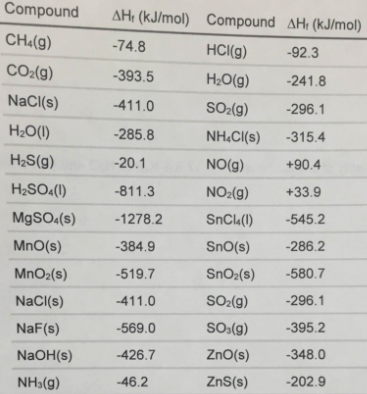Problem: Use a standard enthalpies of formation table to determine the change in enthalpy for each of these reactions.i) CH4(g) + 2 O2(g) → CO2(g) + 2 H2O(l)ii) 2 H2S(g) + 3 O2(g) → 2 H2O(l) + 2 SO2(g)iii) 2 NO(g) + O2(g) → 2 NO2(g)

96% (79 ratings)
Problem Details

Use a standard enthalpies of formation table to determine the change in enthalpy for each of these reactions.

i) CH4(g) + 2 O2(g) → CO2(g) + 2 H2O(l)

ii) 2 H2S(g) + 3 O2(g) → 2 H2O(l) + 2 SO2(g)

iii) 2 NO(g) + O2(g) → 2 NO2(g)What scientific concept do you need to know in order to solve this problem?

Our tutors have indicated that to solve this problem you will need to apply the Enthalpy of Formation concept. You can view video lessons to learn Enthalpy of Formation Or if you need more Enthalpy of Formation practice, you can also practice Enthalpy of Formation practice problems .

What is the difficulty of this problem?

Our tutors rated the difficulty of Use a standard enthalpies of formation table to determine th... as medium difficulty.

How long does this problem take to solve?

Our expert Chemistry tutor, Dasha took 8 minutes to solve this problem. You can follow their steps in the video explanation above.

What professor is this problem relevant for?

Based on our data, we think this problem is relevant for Professor Celan's class at COLLIN.